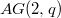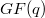﻿

### Matrix forms for isometries of the affine plane$AG(2, q)$ and of the corresponding Miquelian Mobius plane

#### Abstract

We give the matrix form for the isometries of$AG(2, q)$ and the corresponding Miquelian Mobius plane depending on the choice of an irreducible polynomial of second degree over$GF(q)$. In particular we provide the above matrix form for the automorphisms of these planes preserving the set of the affine ellipses of$AG(2,q)$.

DOI Code: 10.1285/i15900932v28n2p175

Keywords: finite affine plane; Miquelian M¨obius plane; squared length; squared distance; isometry

Classification: 05B25

Full Text: PDF